S t a r t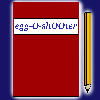Diary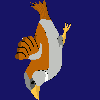Download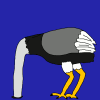Insight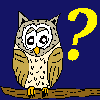Questions?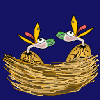Food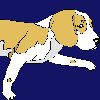Links I m p r i n tKlick hier für die deutschsprachige Version.

 <<<     Conditioned branching     >>> At this column you can learn to create your own egg-O-shOOter. Branches caused by events were exemplified at the previous chapters. Now I would like to explain 'conditioned branching', which means that objects are only activated if other objects exist or not. Let's create a little game: Three eggs are bouncing at the playground and just if the last egg has been hit, our little friend, the fly appears at the scene. We need three eggs and whenever one of the eggs is hit, the fly is activated. This activation is stopped as long as at least one egg is still active. The example looks like this: #1# ObjectNumber=1       #1#Ei Nummer 1 (Gelb) / Egg number 1 (Yellow) ObjectFront=1 ObjectStartActive=1 ObjectTexture=90 ObjectF=H4 ObjectD=H1 ObjectSecMin=-1 ObjectSecMax=-1 ObjectConnect=-1 ObjectKoordPre=-1 ObjectHitDirection=0 ObjectXMin=-800 ObjectXMax=+800 ObjectYMin=-170 ObjectYMax=+200 ObjectDMin=20 ObjectDMax=20 ObjectAMin=0 ObjectAMax=0 ObjectVXMin=100 ObjectVXMax=100 ObjectVYMin=100 ObjectVYMax=200 ObjectVRotMin=100 ObjectVRotMax=200 ObjectRotAxis=3 ObjectVGrowMin=0 ObjectVGrowMax=0 ObjectGravMin=0 ObjectGravMax=0 ObjectMoveXMin=-800 ObjectMoveXMax=+800 ObjectMoveYMin=-200 ObjectMoveYMax=+200 ObjectMoveAMin=-360 ObjectMoveAMax=+360 ObjectMoveRMin=1 ObjectMoveRMax=1000 ObjectMoveType=3 ObjectType=3 ObjectBenefitMin=*0,*0,*0,*0,*0,*0,*0,*0,*0,*0,*0,*0,*0 ObjectBenefitMax=*0,*0,*0,*0,*0,*0,*0,*0,*0,*0,*0,*0,*0 ObjectMirror=0 ObjectMovePointing=0 ObjectHitSound= ObjectAppearSound= ObjectHitTextEnglish= ObjectHitTextGerman= #1# ObjectNumber=2       #1#Ei Nummer 2 (Grau) / Egg number 2 (Grey) ObjectFront=1 ObjectStartActive=1 ObjectTexture=94 ObjectF=H4 ObjectD=H2 ObjectSecMin=-1 ObjectSecMax=-1 ObjectConnect=-1 ObjectKoordPre=-1 ObjectHitDirection=0 ObjectXMin=-800 ObjectXMax=+800 ObjectYMin=-170 ObjectYMax=+200 ObjectDMin=20 ObjectDMax=20 ObjectAMin=0 ObjectAMax=0 ObjectVXMin=100 ObjectVXMax=100 ObjectVYMin=100 ObjectVYMax=200 ObjectVRotMin=100 ObjectVRotMax=200 ObjectRotAxis=3 ObjectVGrowMin=0 ObjectVGrowMax=0 ObjectGravMin=0 ObjectGravMax=0 ObjectMoveXMin=-800 ObjectMoveXMax=+800 ObjectMoveYMin=-200 ObjectMoveYMax=+200 ObjectMoveAMin=-360 ObjectMoveAMax=+360 ObjectMoveRMin=1 ObjectMoveRMax=1000 ObjectMoveType=3 ObjectType=3 ObjectBenefitMin=*0,*0,*0,*0,*0,*0,*0,*0,*0,*0,*0,*0,*0 ObjectBenefitMax=*0,*0,*0,*0,*0,*0,*0,*0,*0,*0,*0,*0,*0 ObjectMirror=0 ObjectMovePointing=0 ObjectHitSound= ObjectAppearSound= ObjectHitTextEnglish= ObjectHitTextGerman= #1# ObjectNumber=3       #1#Ei Nummer 3 (Rot) / Egg number 3 (Red) ObjectFront=1 ObjectStartActive=1 ObjectTexture=98 ObjectF=H4 ObjectD=H3 ObjectSecMin=-1 ObjectSecMax=-1 ObjectConnect=-1 ObjectKoordPre=-1 ObjectHitDirection=0 ObjectXMin=-800 ObjectXMax=+800 ObjectYMin=-170 ObjectYMax=+200 ObjectDMin=20 ObjectDMax=20 ObjectAMin=0 ObjectAMax=0 ObjectVXMin=100 ObjectVXMax=100 ObjectVYMin=100 ObjectVYMax=200 ObjectVRotMin=100 ObjectVRotMax=200 ObjectRotAxis=3 ObjectVGrowMin=0 ObjectVGrowMax=0 ObjectGravMin=0 ObjectGravMax=0 ObjectMoveXMin=-800 ObjectMoveXMax=+800 ObjectMoveYMin=-200 ObjectMoveYMax=+200 ObjectMoveAMin=-360 ObjectMoveAMax=+360 ObjectMoveRMin=1 ObjectMoveRMax=1000 ObjectMoveType=3 ObjectType=3 ObjectBenefitMin=*0,*0,*0,*0,*0,*0,*0,*0,*0,*0,*0,*0,*0 ObjectBenefitMax=*0,*0,*0,*0,*0,*0,*0,*0,*0,*0,*0,*0,*0 ObjectMirror=0 ObjectMovePointing=0 ObjectHitSound= ObjectAppearSound= ObjectHitTextEnglish= ObjectHitTextGerman= #1# ObjectNumber=4       #1#Fliege / Fly ObjectFront=1 ObjectStartActive=0 ObjectTexture=11/50,12/50 ObjectF=-1 ObjectD=-1 ObjectSecMin=-1 ObjectSecMax=-1 ObjectConnect=-1 ObjectKoordPre=c1,c2,c3,1,2,3 ObjectHitDirection=0 ObjectXMin_0 ObjectXMax_0 ObjectYMin_0 ObjectYMax_0 ObjectDMin=20 ObjectDMax=20 ObjectAMin=0 ObjectAMax=0 ObjectVXMin=100 ObjectVXMax=100 ObjectVYMin=100 ObjectVYMax=200 ObjectVRotMin=100 ObjectVRotMax=200 ObjectRotAxis=3 ObjectVGrowMin=0 ObjectVGrowMax=0 ObjectGravMin=0 ObjectGravMax=0 ObjectMoveXMin=-800 ObjectMoveXMax=+800 ObjectMoveYMin=-200 ObjectMoveYMax=+200 ObjectMoveAMin=-360 ObjectMoveAMax=+360 ObjectMoveRMin=1 ObjectMoveRMax=1000 ObjectMoveType=3 ObjectType=3 ObjectBenefitMin=*0,*0,*0,*0,*0,*0,*0,*0,*0,*0,*0,*0,*0 ObjectBenefitMax=*0,*0,*0,*0,*0,*0,*0,*0,*0,*0,*0,*0,*0 ObjectMirror=0 ObjectMovePointing=0 ObjectHitSound= ObjectAppearSound= ObjectHitTextEnglish= ObjectHitTextGerman= To keep the overview it is a good idea to set the variables BunniesMin=0 BunniesMax=0 ... JumpingBunniesMin=0 JumpingBunniesMax=0 to deactivate all egg throwing bunnies and all jumpingbunnys. To stop the activation of the fly as long as one of the given eggs is active you must use the variable ObjectKoordPre. All object numbers listed with the character 'c' in front inhibit the activation of object number 4 (the fly), as long as one of them is still active. Only if object 1, 2 and 3 are deactivated (by a hit), the fly appears - assuming that an event is triggered who activates the fly. Because of the fact that this activation of the fly is done at each hit of an egg, it will appear unavoidable as soon as the last egg is hit. The objects 1 to 3 are additionally the objects for the adoption of the coordinates and so the fly appears at the point where the last egg was hit. It looks like the fly comes out of the last egg. Everything alright? That wasn't too tough. Let's realise the condition 'fly appears only if the yellow egg is still active' This kind of condition is realised by the character 'b' in front of the object number. Set ObjectKoordPre=b1,2,3 at the fly and set ObjectF=-1 at the yellow egg. Shoot firstly at the yellow egg and then at the grey and red egg - the fly doesn't appear at the scene. Restart the level (or press 'N'-key) and shoot at the red or grey egg - the fly will appear immediately, because the yellow egg is still active. Not very nice is the fact that the fly doesn't necessarily come out of the hit egg. If you shoot at the red egg first, the fly appears at the point of the grey egg. To correct that, the character 'g' is useful. Therewith it is proofed, if the object listed at ObjectKoordPre is the same object that triggered the event and if so, the coordinates are assumed. By setting ObjectKoodrPre=b1,g2,g3 the fly always comes out of the hit egg, as soon as the yellow egg is still active.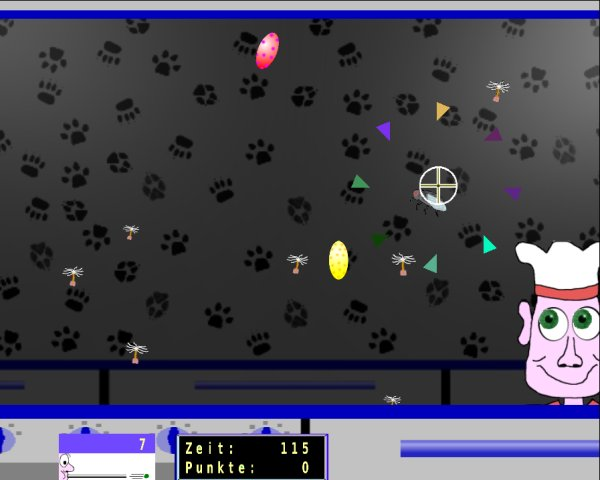Note that the sequence of ObjectKoordPre is important! No coordinates are assumed of objects with the character 'b', 'c' or 'd' in front! Those object numbers just define the conditions. The eradication of the sequence is aborted, as soon as an active object for assuming coordinates is found. If you set e.g. ObjectKoordPre=1,2,b3 and object number 1 is active, the sequence is aborted before object 2 is reached. The testing if object number 3 exists is NOT executed! There may be the following reasons why NO active object for assuming coordinates can be found: 1.) You did not define an object for assuming coordinates (ObjectKoordPre=-1) or there are just 'conditional objects' listed ('b', 'c' oder 'd'). The object is activated with it's own given coordinates. 2.) You defined one or more objects for assuming coordinates, but none of them is active. The activation will be STOPPED in this case!